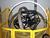## Entering a character value in RPGLETags:
AS/400
RPGLE
If I enter a character value of '12345' then I need to get a output of 123.45 . I did like %dec(var:5:2) but its getting a run time error.

Thanks. We'll let you know when a new response is added.

## Discuss This Question: 2 Replies

Thanks. We'll let you know when a new response is added.
•%Dec converts the string variable to a decimal using the decimal point in the string.  It makes the result second parameter wide and third parameter decimal points.

Your example 12345 with 2 additional decimal points would require a width of 5+2 or 7

%dec(var:7:2)  would be 12345.00

to get what you want you might do this

%dec(var:7:2)/100  would be 123.45

or

%dec((%substr(var:1:3) + '.' + %substr(val:4:2)):5:2)  would be 123.45

report
• Thank you..
report